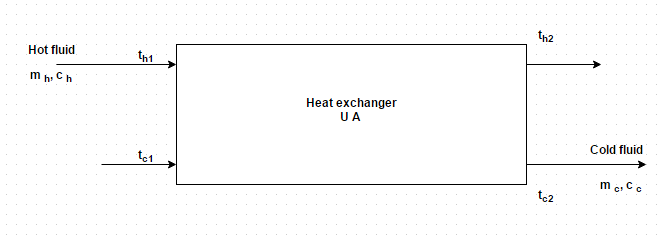Q.1
##### When two fluids of the heat exchanger are separated by a plane wall, the thermal resistance comprises
• a) i and ii
• b) i, ii and iii
• c) ii and iii
• d) i and iii
Q.2
##### Figure represents the block diagram of a heat exchanger. There were some aspects in the design and performance analysis of a heat exchanger. Identify the correct one• a) The coolant picks up heat = m h c c (t c1 – t c2)
• b) The hot fluid gives up heat = m h c h (t h1 – t c2)
• c) The coolant picks up heat = m c c h (t h1 – t c2)
• d) The hot fluid gives up heat = m h c h (t h1 – t h2)
Q.3
##### The heat loss from unpainted aluminum side of a house has been calculated on the presumption that overall coefficient of heat transfer is 5 W/m 2 K. Later, it was discovered that the air pollution levels are such that fouling factor on this side is of the order of 0.mK/W. Find overall heat transfer coefficient of dirty side
• a) 2.9875 W/m2 K
• b) 3.9875 W/m2 K
• c) 4.9875 W/m2 K
• d) 5.9875 W/m2 K
Q.4
##### What is the value of fouling factor for engine exhaust?
• a) 0.002 m2 K/W
• b) 0.003 m2 K/W
• c) 0.004 m2 K/W
• d) 0.005 m2 K/W
Q.5
##### What is the value of fouling factor for industrial liquids?
• a) 0.0004 m2 K/W
• b) 0.0003 m2 K/W
• c) 0.0002 m2 K/W
• d) 0.0001 m2 K/W
Q.6
##### After being in service for a period of six months, a heat exchanger transformsless heat than it does what new. Determine the effective fouling factor in terms of its clean overall heat transfer coefficient. It may be presumed that the heat exchanger operates between the same temperature differentials and that there is no change in the effective surface area due to scale build up
• a) 0.13/ U CLEAN
• b) 0.11/ U CLEAN
• c) 0.09/ U CLEAN
• d) 0.07/ U CLEAN
Q.7
##### In a counter flow heat exchanger, water flowing through a tube ofcm inner diameter is heated by steam condensing on the outside of the tube. The convective film coefficient on the water and steam side are estimated to beandk J/m2 hr degree. Neglecting tube thickness and its resistance to heat flow, workout the overall heat transfer coefficient for the heat exchanger
• a) 4500 k J/m2 hr degree
• b) 5500 k J/m2 hr degree
• c) 6500 k J/m2 hr degree
• d) 7500 k J/m2 hr degree
Q.8
##### A heat exchanger to preheat oil for a furnace was designed without considering the possibility of scale formation, and the overall heat transfer coefficient based on the fuel oil side wask J/m2 hr degree. What would be the corrected coefficient of heat transfer if a fouling factor of 0.m2 hr degree/k J for the fuel oil is taken into account?
• a) 1777.78 k J/m2 hr degree
• b) 1666.78 k J/m2 hr degree
• c) 1555.78 k J/m2 hr degree
• d) 1444.78 k J/m2 hr degree
Q.9
##### A copper pipe (k =W/m K) having inner diameter of 1.cm and 2.0 cm outside diameter conveys water and the oil flows through the annular passage between this pipe and a steel pipe. On the water side, the film coefficient isW/m2 K and the fouling factor is 0.m2 K/W. The corresponding values for the oil side areW/m2 K and 0.m2 K/W. Estimate the overall heat transfer coefficient between the oil and water
• a) 235.16 W/m2 K
• b) 335.16 W/m2 K
• c) 435.16 W/m2 K
• d) 535.16 W/m2 K
Q.10
##### Unit of fouling factor is
• a) m2 K/kcal
• b) m2 hr K/kcal
• c) m2 hr/kcal
• d) m2 hr K